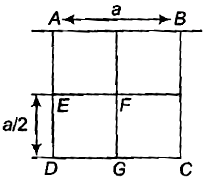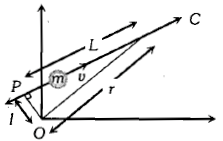From a uniform square plate of side a and mass m, a square portion DEFG of side $\frac{\mathrm{a}}{2}$ is removed. Then, the moment of inertia of the remaining portion about the axis AB is1.

2.

3.

4.

Concept Questions :-

Moment of inertia
High Yielding Test Series + Question Bank - NEET 2020

Difficulty Level:

Two bodies having masses ${m}_{1}$ and ${m}_{2}$ and velocities ${u}_{1}$ and ${u}_{2}$ collide form a composite system. If ${m}_{1}$${u}_{1}$ + ${m}_{2}$${u}_{2}$ = (${m}_{1}$ $\ne$${m}_{2}$), the velocity of composite system will be

1.  ${u}_{1}$ - ${u}_{2}$

2.  ${u}_{1}$ + ${u}_{2}$

3.

4.  Zero

Concept Questions :-

Linear momentum
High Yielding Test Series + Question Bank - NEET 2020

Difficulty Level:

Centre of the mass of 3 particles 10 kg, 20kg, and 30kg is at (0.0,0). Where should a particle of mass 40 kg be placed so that the combined center of mass will be at (3, 3, 3)?

1.  (0, 0, 0)

2.  (7, 5, 7, 5, 7, 5)

3.  (1, 2, 3)

4.  (4, 4, 4)

Concept Questions :-

Center of mass
High Yielding Test Series + Question Bank - NEET 2020

Difficulty Level:

The position of a particle is given by:  and momentum . The angular momentum is perpendicular to

1.  X-axis

2.  Y-axis

3.  Z-axis

4.  Line at equal angles to all the three axes

Concept Questions :-

Angular momentum
High Yielding Test Series + Question Bank - NEET 2020

Difficulty Level:

A body falling vertically downward under gravity breaks into two parts of unequal messes. The center of mass of the parts taken together shifts horizontally towards

1.  heavier piece

2.  lighter piece

3.  does not shift horizontally

4.  depends on the vertical velocity at the time of breaking

Concept Questions :-

Center of mass
High Yielding Test Series + Question Bank - NEET 2020

Difficulty Level:

A particle of mass m moves along line PC with velocity v as shown. What is the angular momentum of the particle about O1.  mvL

2.  mvl

3.  mvr

4.  0

Concept Questions :-

Angular momentum
High Yielding Test Series + Question Bank - NEET 2020

Difficulty Level:

A solid sphere, disc, and solid cylinder all Of the same mass and made up Of same material are allowed to roll down (from rest) on an inclined plane, then

1.  Solid sphere reaches the bottom first

2.  Solid sphere reaches the bottom late

3.  The disc will reach the bottom first

4.  All of them reach the bottom at the same time

Concept Questions :-

Rolling motion
High Yielding Test Series + Question Bank - NEET 2020

Difficulty Level:

If the angular momentum of a rotating body about a fixed axis is increased by 10%, its kinetic energy will be increased by

(1) 10%                  (2) 20%              (3) 21%                       (4) 5%

High Yielding Test Series + Question Bank - NEET 2020

Difficulty Level:

A cylinder of 500 g and radius 10 cm has moment of inertia (about its natural axis)

(1)

(2)

(3)

(4)

Concept Questions :-

Moment of inertia
High Yielding Test Series + Question Bank - NEET 2020

Difficulty Level:

Two rings of the same radius and mass are placed such that their centers are at a common point and their planes are perpendicular to each other. The moment of inertia of the system about an axis passing through the center and perpendicular to the plane of one of the rings is (mass of the ring and radius = r)

1.  $\frac{1}{2}{\mathrm{mr}}^{2}$

2.  ${\mathrm{mr}}^{2}$

3.  $\frac{3}{2}{\mathrm{mr}}^{2}$

4.  $2{\mathrm{mr}}^{2}$

Concept Questions :-

Moment of inertia